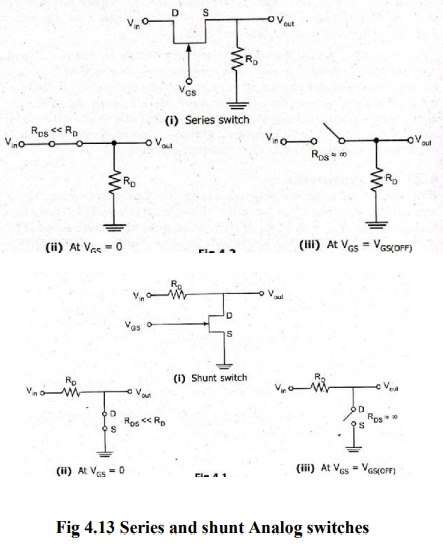Home | | Linear Integrated Circuits | A to D Converter- Specifications

# A to D Converter- Specifications

Like DAC, ADCs are also having many important specifications.

A to D Converter- Specifications

Like DAC, ADCs are also having many important specifications. Some of them are Resolution, Quantization error, Conversion time, Analog error, Linearity error, DNL error, INL error & Input voltage range.

### Resolution:

The resolution refers to the finest minimum change in the signal which is accepted for conversion, and it is decided with respect to number of bits. It is given as 1/2n, where ‘n’ is the number of bits in the digital output word. As it is clear, that the resolution can be improved by increasing the number of bits or the number of bits representing the given analog input voltage.

Resolution can also be defined as the ratio of change in the value of input voltage Vi, needed to change the digital output by 1 LSB. It is given as

Resolution = ViFS / (2n – 1)

Where ‘ViFS’ is the full-scale input voltage.

‘n’ is the number of output bits.

### Quantization error:

If the binary output bit combination is such that for all the values of input voltage Vi between any two voltage levels, there is a unavoidable uncertainty about the exact value of Vi when the output is a particular binary combination. This uncertainty is termed as quantization error. Its value is ± (1/2) LSB. And it is given as,

QE = ViFS / 2(2n – 1)

Where ‘ViFS’ is the full-scale input voltage

‘n’ is the number of output bits.

Maximum the number of bits selected, finer the resolution and smaller the quantization error.

### Conversion Time:

It is defined as the total time required for an A/D converter to convert an analog signal to digital output. It depends on the conversion technique and propagation delay of the circuit components.

### Analog error:

An error occurring due to the variations in DC switching point of the comparator, resistors, reference voltage source, ripples and noises introduced by the circuit components is termed as Analog error.

### Linearity Error:

It is defined as the measure of variation in voltage step size. It indicates the difference between the transitions for a minimum step of input voltage change. This is normally specified as fraction of LSB.

### Differential Non-Linearity(DNL) Error:

The analog input levels that trigger any two successive output codes should differ by 1 LSB. Any deviation from this 1 LSB value is called as DNL error.

### Integral Non-Linearity (INL) Error:

The deviation of characteristics of an ADC due to missing codes causes INL error. The maximum deviation of the code from its ideal value after nulling the offset and gain errors is called as Integral Non-Linearity Error.

### Input Voltage Range:

It is the range of voltage that an A/D converter can accept as its input without causing any overflow in its digital output.

### Analog Switches

There were two types of analog switches. Series and Shunt switch. The Switch operation is shown for both the cases VGS=0 VGS= VGs (off)Tags : Analog to Digital Converters , Linear Integrated Circuits : Analog to Digital And Digital to Analog Converters
Study Material, Lecturing Notes, Assignment, Reference, Wiki description explanation, brief detail
Linear Integrated Circuits : Analog to Digital And Digital to Analog Converters : A to D Converter- Specifications | Analog to Digital Converters